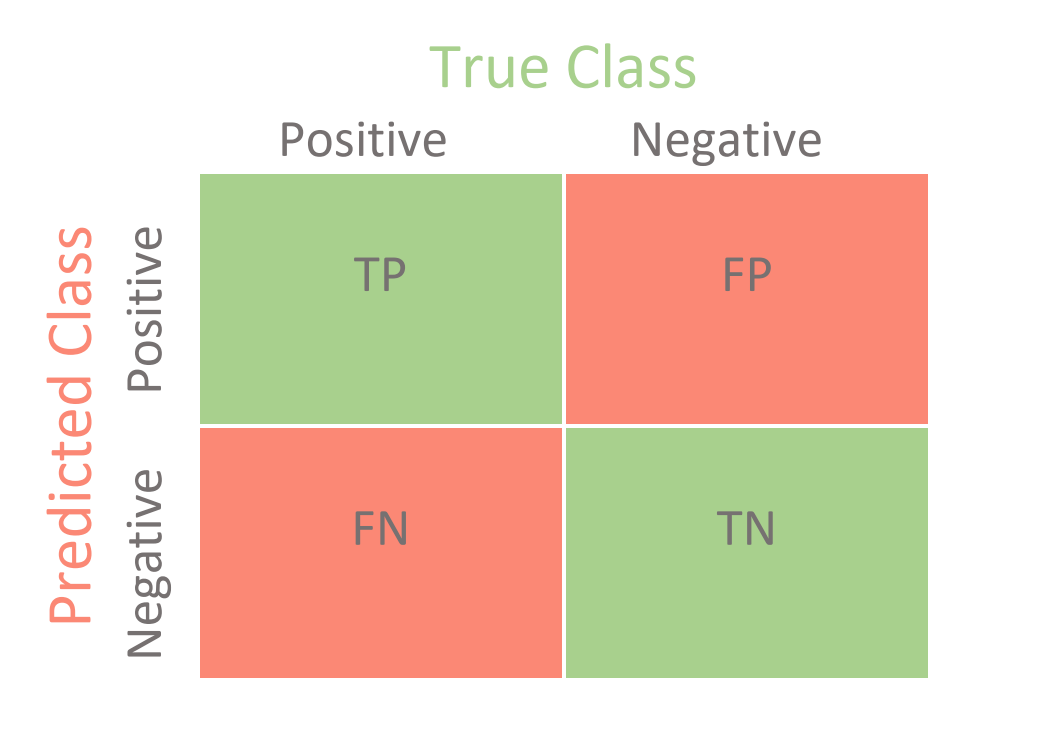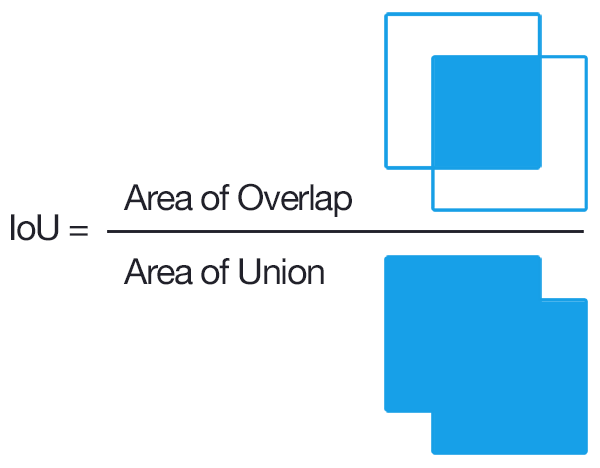This article was published as a part of the Data Science Blogathon

Deep learning techniques like image classification, segmentation, object detection are used very commonly. Choosing the right evaluation metrics is very crucial to decide which model to use, how to tune the hyperparameters, the need for regularization techniques, and so on. I have included the metrics I have used to date.

## Classification Metrics

Let’s first consider Classification metrics for image classification. Image classification problems can be binary or multi-classification. Example for binary classification includes detection of cancer, cat/dog, etc. Some examples for Multi-label classification include MNIST, CIFAR, and so on.

The first metric that you think of usually is Accuracy. It’s a simple metric that calculates the ratio of Correct predictions to wrong predictions. But is it always valid?

Let’s take a case of an imbalanced dataset of cancer patients. Here, the majority of the data points will belong to the negative class and very few in the positive class. So, just by classifying all the patients as “Negative”, the model would have achieved great accuracy!

### Confusion Matrix

The next step usually is to plot the confusion Matrix.  It has 4 categories: True positives, True negatives, false positives, and false negatives. Using this matrix, we can calculate various useful metrics!

Accuracy =  (TP + TN) / ( TP + TN + FP + FN)You can find this using just a few lines of code with sklearn metrics library.

`from sklearn.metrics import confusion_matrix, accuracy_score`
```# Threshold can be optimized for each problem
threshold=0.5
preds_list = preds_list >= threshold
tn, fp, fn, tp = confusion_matrix(labels_list, preds_list).ravel()
accuracy = accuracy_score(labels_list, preds_list```

You would have probably heard terms like recall or sensitivity. They are the same!

• #### Sensitivity/ True Positive Rate:

TPR/Sensitivity denotes the percentage/fraction of the positive class that was correctly predicted and classified! It’s also called Recall.

Sensitivity = True Positives/ (True Positives + True Negatives)

An example: What percent of actual cancer-infected patients were detected by the model?

• #### Specificity / True Negative Rate:

While it’s essential to correctly predict positive class, imagine what would happen if a cancer-negative patient has been told incorrectly that he’s in danger! (False positive)

Specificity is a metric to calculate what portion of the negative class has been correctly predicted and classified.

Specificity = True Negatives/ (False Positives + True Negatives)

This is also called as True Negative Rate (TPR)

Specificity and Sensitivity are the most commonly used metrics. But, we need to understand FPR also to get ROC.

• #### False Positive Rate

This calculates how many negative class samples were incorrectly classified as positive.

FPR = False Positives / (False Positives + True Negatives)
FPR = 1 – Specificity

For a good classification model, what is that we desire?

A higher TPR and lower FPR!

Another useful method is to get the AUC ROC curve for your confusion matrix. Let’s look into it!

• #### AUC ROC Curve

ROC stands for Receiver Operator Characteristic (ROC). AUC just means Area under the curve. Here, we plot the True Positive Rate against False Positive Rate for various thresholds.

Generally, if a prediction has a value above 0.5, we classify it into positive class, else, negative class. Here, this deciding boundary 0.5 is denoted as the threshold. It’s not always necessary to use 0.5 as the threshold, sometimes other values might give the best results. To find out this, we plot TPR  vs  FPR against a range of threshold values. Usually, the thresholds are varied from 0.1, 0.2, 0.3, 0.4, and so on to 1.Image: Source

For a particular threshold, if you want to calculate a ROC AUC Score, sklearn provides a function. You can use it as shown.

```from sklearn.metrics import roc_auc_score
roc_auc = roc_auc_score(labels, predictions)```

The top left corner of the graph is where you should look for your optimal threshold!

If you want to plot the ROC AUC graph, you can use blow snippets

```fpr, tpr, thresholds = metrics.roc_curve(y, scores, pos_label=2)
import matplotlib.pyplot as plt
plt.plot(fpr,tpr)
plt.show()```

Here, the fpr and tpr is given by the function will be a list/array containing the respective values for each threshold value in the list.

You can also plot sensitivity and specificity against thresholds to get more information.

## Object detection Metrics

Object detection has many applications including face detection, Lane detection in Auto-driver systems, and so on. Here, we need to use a different set of metrics to evaluate. The most popular one is IOU. Let’s begin!

• #### IOU (Intersection over Union)

So in object detecting or segmentation problems, the ground truth labels are masks of a portion or a bounding box where the object is present. The IOU metric finds the difference between the prediction bounding box and the ground truth bounding box.

IOU = Area of Intersection of the two bounding boxes / Area of UnionSource: Image

IOU will be a value between 0-1. For perfectly overlapping boxes, it will be 1 and 0 for non-overlapping prediction. Generally, IOU should be above 0.5 for a decent object detection model.

• #### Mean Average Precision (mAP)

Using the IOU, precision, and recall can be calculated.

How?

You have to set an IOU Threshold value. For example, let’s say I keep the IOU threshold as 0.5. Then for a prediction of IOU as 0.8, I can classify it as True positive. If it’s 0.4 (less than 0.5) then it is a False Positive.  Also note that if we change the threshold to 0.4, then this prediction would classify as True Positive. So, varying thresholds can give different metrics.

Next, Average Precision (AP) is obtained by finding the area under the precision-recall curve. The mAP for object detection is the average of the AP calculated for all the classes to determine the accuracy of a set of object detections from a model when compared to ground-truth object annotations of a dataset.

The mean Average Precision is calculated by taking the mean of AP over all classes and/or overall IoU thresholds.

Many object detection algorithms including Faster R-CNN, MobileNet use this metric. This metric provides numerical value making it easier to compare with other models.

Thanks for reading! You can connect with me at [email protected]

The media shown in this article on Metrics for Image Classification are not owned by Analytics Vidhya and are used at the Author’s discretion.